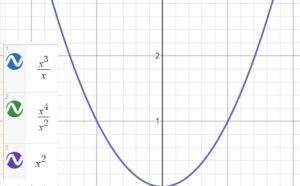# Identical Function (Equal Function)

## What is an Identical Function?

An identical function has a matching “twin” function. Their graphs overlap completely.

More formally, two functions are identical (or equal) if

1. Both functions have equal domains,
2. Both functions have equal ranges,
3. The functions are equal for all values in the domain.

Although two identical functions always have overlapping graphs, the converse isn’t always true; You can’t simply graph two functions are conclude they are identical because their graphs overlap. It is possible for two functions to look the same on a graph, yet not meet the stringent requirements for an identical function. As an example, the graphs of x3/x, x4/x2 and x 2 overlap:However, they are not identical because they do not have the same domains:

• x3/x and x4/x2 have domains of the set of non-zero real numbers,
• x 2 has a domain of the set of real numbers.

In other words, you’ll find a hole at zero of the first two functions that doesn’t exist on the third. This is practically impossible to see on a standard graph, which is why it’s so important to compare domains and ranges.

## Identical Function in R

In R, the identical(x, y) function tests exact equality in if and while statements or logical expressions using && or ||.

Usage (R Documentation):

identical(x, y, num.eq = TRUE, single.NA = TRUE, attrib.as.set = TRUE, ignore.bytecode = TRUE, ignore.environment = FALSE, ignore.srcref = TRUE)

## References

Graphs created on Desmos.com.
R Documentation. identical. Retrieved November 10, 2020 from: https://www.rdocumentation.org/packages/base/versions/3.6.2/topics/identical

CITE THIS AS:
Stephanie Glen. "Identical Function (Equal Function)" From StatisticsHowTo.com: Elementary Statistics for the rest of us! https://www.statisticshowto.com/identical-function/